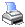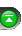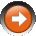NinjaScript > Language Reference > Indicator > Add()Definition
Adds plot and line objects that define how an indicator data series is visualized on a chart. When this method is called to add a plot, an associated DataSeries object is created that is held in the Values collection.

Syntax

Parameters

 plot A Plot object line A Line object

Examples

 // Adds a blue line style plot Add(new Plot(Color.Blue, "myPlot"));   // Adds a blue historgram style plot Add(new Plot(Color.Blue, PlotStyle.Bar, "myPlot"));   // Adds a blue line style plot with a thickness of 3 Add(new Plot(new Pen(Color.Blue, 3), "myPlot"))   // Adds an oscillator line at a value of 30 Add(new Line(Color.Gray, 30, "Lower"));

Tips

 1 There are many variations of defined plot and line objects that are not documented. We suggest using the NinjaScript indicator wizard to generate your indicator plot and oscillator lines.
 2 Plot objects DO NOT hold the actual indicator values. They simply define how the indicator's values are plotted on a chart.
 3 An indicator may calculate multiple values and therefore hold multiple plots to determine the display of each calculated value. Indicator values are held in the indicator's Values collection.
 4 If your indicator calculates and stores three values then plots are assigned as per the example below.

 protected override void Initialize() {     // Add three plots and associated DataSeries objects     Add(new Plot(Color.Blue, "PlotA")); // Defines the plot for Values     Add(new Plot(Color.Red, "PlotB")); // Defines the plot for Values     Add(new Plot(Color.Green, "PlotC")); // Defines the plot for Values }